Python Tuples Tutorial with Examples

View more Tutorials: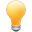Follow us on our fanpages to receive notifications every time there are new articles.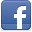Facebook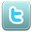Twitter

1- Python Tuples

In Python, Tuples is a sequence of values containing many elements, which is similar to List. Unlike List, Tuples is an immutable data type, all updates on the Tuple create a new entity on the memory.
In order to write a Tuple, you need to put the elements into parentheses ( ) and separated by comma. These elements of the list are indexed with the starting index 0.
tupleExample.py

fruitTuple = ("apple", "apricot", "banana","coconut", "lemen")
otherTuple = (100, "one", "two", 3)
print ("Fruit Tuple:")
print (fruitTuple)
print (" --------------------------- ")
print ("Other Tuple:")
print (otherTuple)
Output:

Fruit Tuple:
('apple', 'apricot', 'banana', 'coconut', 'lemen')
---------------------------
Other List:
(100, 'one', 'two', 3)

2- List vs Tuple

See more:
List and Tuple are a sequence of elements. There are some following differences between them:
• Writing a list, you need to use square brackets [] while writing a Tuple, you use parentheses ( ).

# This is a Tuple.
aTuple = ("apple", "apricot", "banana")

# This is a List.
aList =  ["apple", "apricot", "banana"]
• List is a mutable data type, you can use the append() method to add the element to List, or use the remove() method to remove elements from List without creating another "List" entity in memory.
listMemoryTest.py

list1 =  [1990, 1991, 1992]
print ("list1: ", list1)

# Address of list1 in the memory.
list1Address = hex ( id(list1) )
print ("\n")
print ("Append element 2001 to list1")

# Append a element to list1.
list1.append(2001)
print ("list1 (After append): ", list1)

# Address of list1 in the memory.
list1Address = hex ( id(list1) )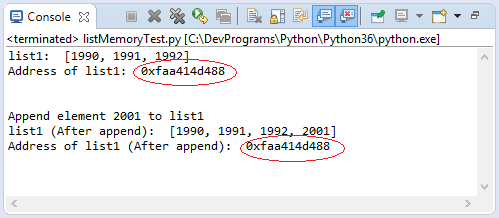• Tuple is an immutable object, it does not have the methods append (), remove (),... like list. You may think that some methods, or operators are used to update Tuple but the fact that it is based on the original Tuple to create a new Tuple.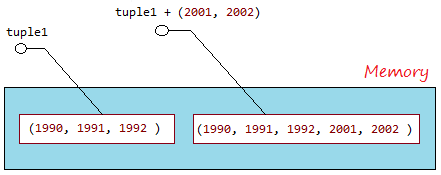tupleMemoryTest.py

tuple1 =  (1990, 1991, 1992)

# Address of tuple1 in the memory.
tuple1Address = hex ( id(tuple1) )

# Concatenate a tuple to tuple1.
tuple1 = tuple1 + (2001, 2002)

# Address of tuple1 in the memory.
tuple1Address = hex ( id(tuple1) )
Output:

Address of tuple1 (After concat): 0x9096778f10

3- Access the elements of Tuples

Access the elements
Use the 'for' loop to access the elements of Tuple:
elementAccessExample.py

fruits = ("apple", "apricot", "banana", "coconut", "lemen", "plum", "pear")

for fruit in fruits :
print ("Fruit: ", fruit)
Output:

Fruit: apple
Fruit: apricot
Fruit: banana
Fruit: coconut
Fruit: lemen
Fruit: plum
Fruit: pear
Access via index:
You can also access the elements of Tuple via index. The elements of Tuple are indexed from  left to right, starting at 0.
indexAccessExample.py

fruits = ("apple", "apricot", "banana", "coconut", "lemen", "plum", "pear")
print ( fruits )

# Element count.
print ("Element count: ", len(fruits) )

for i in range (0, len(fruits) ) :
print ("Element at ", i, "= ", fruits[i] )

# A sub Tuple of elements with index from 1 to 4 (1, 2, 3)
subTuple = fruits[1: 4]
# ('apricot', 'banana', 'coconut')
print ("Sub Tuple [1:4] ", subTuple )
Output:

('apple', 'apricot', 'banana', 'coconut', 'lemen', 'plum', 'pear')
Element count: 7
Element at 0 = apple
Element at 1 = apricot
Element at 2 = banana
Element at 3 = coconut
Element at 4 = lemen
Element at 5 = plum
Element at 6 = pear
Sub Tuple [1:4]  ('apricot', 'banana', 'coconut')
You can also access the elements of Tuple via negative index, the elements are indexed from right to left with the values -1, -2,...
indexAccessExample2.py

fruits = ("apple", "apricot", "banana", "coconut", "lemen", "plum", "pear")
print ( fruits )
print ("Element count: ", len(fruits) )

print ("fruits[-1]: ", fruits[-1])
print ("fruits[-2]: ", fruits[-2])
subTuple1 = fruits[-4: ]

print ("\n")
print ("Sub Tuple fruits[-4: ] ")
print (subTuple1)
subTuple2  = fruits[-4:-2]
print ("\n")
print ("Sub Tuple fruits[-4:-2] ")
print (subTuple2)
Output:

('apple', 'apricot', 'banana', 'coconut', 'lemen', 'plum', 'pear')
Element count: 7
fruitList[-1]:  pear
fruitList[-2]:  plum

Sub Tuple fruits[-4: ]
('coconut', 'lemen', 'plum', 'pear')

Sub Tuple fruits[-4:-2]
('coconut', 'lemen')

4- Update Tuples

Note that Tuple is immutable, so it only has methods or operators to access, or create a new Tuple from the original Tuple.
updateTupleExample.py

tuple1 = (1990, 1991, 1992)
print ("tuple1: ", tuple1)
print ("Concat (2001, 2002) to tuple1")

tuple2 = tuple1 + (2001, 2002)
print ("tuple2: ", tuple2)

# A sub Tuple, containing elements from index 1 to 4 (1,2,3)
tuple3 = tuple2[1:4]
print ("tuple2[1:4]: ", tuple3)

# A sub Tuple, containing elements from index 1 to the end.
tuple4 = tuple2[1: ]
print ("tuple2[1: ]: ", tuple4)
Output:

tuple1: (1990, 1991, 1992)
Concat (2001, 2002) to tuple1
tuple2: (1990, 1991, 1992, 2001, 2002)
tuple2[1:4]: (1991, 1992, 2001)
tuple2[1: ]: (1991, 1992, 2001, 2002)

5- Operators for Tuples

The same as String, Tuple has 3 operators including +, * , in.
 Operator Description Example + The operator is used to concatenate 2 Tuples to create a new Tuple (1, 2, 3) + ("One","Two") --> (1, 2, 3, "One", "Two") * The operator is used to concatenate multiple copies of the same Tuple. And create a new Tuple (1, 2) * 3 --> (1, 2, 1, 2, 1, 2) in Check whether an element is in a Tuple, which returns True or False. "Abc" in ("One", "Abc") --> True
tupleOperatorsExample.py

tuple1 = (1, 2, 3)
tuple2 = ("One", "Two")
print ("tuple1: ", tuple1)
print ("tuple2: ", tuple2)
print ("\n")

tuple12 = tuple1 + tuple2
print ("tuple1 + tuple2: ", tuple12)
tuple2x3 = tuple2 * 3
print ("tuple2 * 3: ", tuple2x3)
hasThree = "Three" in tuple2
print ("'Three' in tuple2? ", hasThree)
Output:

tuple1: (1, 2, 3)
tuple2: ('One', 'Two')

tuple1 + tuple2: (1, 2, 3, 'One', 'Two')
list2 * 3: ('One', 'Two', 'One', 'Two', 'One', 'Two')
'Three' in tuple2? False

6- Functions for Tuples

Function Description
cmp(list1, list2) Compares elements of both Tuples. This function was removed from Python3.
len(list) Gives the total length of the Tuple.
max(list) Returns item from the Tuple with max value.
min(list) Returns item from the Tuple with min value.
tuple(seq) Converts a List into Tuple.
tupleFunctionsExample.py

tuple1 = (1991, 1994, 1992)
tuple2 = (1991, 1994, 2000, 1992)
print ("tuple1: ", tuple1)
print ("tuple2: ", tuple2)

# Return element count.
print ("len(tuple1): ", len(tuple1) )
print ("len(tuple2): ", len(tuple2) )

# Maxinum value in the Tuple.
maxValue = max(tuple1)
print ("Max value of tuple1: ", maxValue)

# Minimum value in the Tuple.
minValue = min(tuple1)
print ("Min value of tuple1: ", minValue)

# (all)
# List
list3 = [2001, 2005, 2012]
print ("list3: ", list3)

# Convert a List to a tuple.
tuple3 = tuple (list3)
print ("tuple3: ", tuple3)
Output:

tuple1: (1991, 1994, 1992)
tuple2: (1991, 1994, 2000, 1992)
len(tuple1): 3
len(tuple2): 4
Max value of tuple1: 1994
Min value of tuple1: 1991
list3: [2001, 2005, 2012]
tuple3: (2001, 2005, 2012)
See more:

7- Methods

Method Description
tuple.count(obj) Returns count of how many times obj occurs in Tuple
tuple.index(obj, [start, [stop]]) Returns the lowest index in Tuple that obj appears. Raises ValueError if the value not found.

If there are parameters start, stop, just search from start to stop index (And not included stop).
Example:
tupleMethodsExample.py

years =  ( 1990 ,  1991 ,  1993 ,  1991 ,  1993 ,  1993 ,  1993 )
print ("Years: ", years)
print ("\n")

# return number of occurrences of 1993
print ("years.count(1993): ",years.count(1993) )

# Find the index that appears 1993
print ("years.index(1993): ", years.index(1993) )

# Find the index that appears 1993, from index 3
print ("years.index(1993, 3): ", years.index(1993, 3) )

# Find the index that appears 1993, from index 4 to 5 (Not included 6)
print ("years.index(1993, 4, 6): ", years.index(1993, 4, 6) )
Output:

Years: (1990, 1991, 1993, 1991, 1993, 1993, 1993)

years.count(1993): 4
years.index(1993): 2
years.index(1993, 3): 4
years.index(1993, 4, 6): 4
See more:

View more Tutorials:

Maybe you are interested

These are online courses outside the o7planning website that we introduced, which may include free or discounted courses.

•Python 3 For Beginner - Object-Oriented Programming
•Complete Python Web Course: Build 8 Python Web Apps
•Master Computer Vision™ OpenCV4 in Python with Deep Learning
•Ultimate Coding Course for Web App and Android Development
•Learn iPython: The Full Python IDE
•Python GUI : From A-to-Z With 2 Final Projects
•Learning iPython Notebook
•CSS Development (with CSS3!)
•Python Fundamentals
•Python MySql From Scratch
•Basic Concepts of Web Development, HTTP and Java Servlets
•Python Programming Full Course (Basics,OOP,Modules,PyQt)
•Learning Path: Python GUI Projects
•Twitter Bootstrap in Responsive Web Design
•Learning Path: Python: Design and Architect Python Apps
•Backup and Restore Fundamentals in PostgreSQL DB - Level 2
•Python 3000: Tactical SQL Quick-Start
•Python BeautifulSoup: Extract Web Data Beautifully
•Python 3000: Tactical File I/O
•Web Programming with Python
•Learning Path: Python Web Development
•Learn Programming in Python With the Power of Animation
•New to Python Automation..?Try Step by Step Python 4 Testers
•*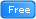* Introduction to Ruby on Rails
•Python 2000: Beyond The Basics